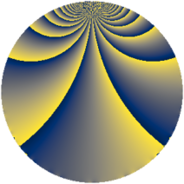# Properties

 Label 536.1.baLevel $536$ Weight $1$ Character orbit 536.ba Rep. character $\chi_{536}(19,\cdot)$ Character field $\Q(\zeta_{66})$ Dimension $20$ Newform subspaces $1$ Sturm bound $68$ Trace bound $0$

# Learn more

## Defining parameters

 Level: $$N$$ $$=$$ $$536 = 2^{3} \cdot 67$$ Weight: $$k$$ $$=$$ $$1$$ Character orbit: $$[\chi]$$ $$=$$ 536.ba (of order $$66$$ and degree $$20$$) Character conductor: $$\operatorname{cond}(\chi)$$ $$=$$ $$536$$ Character field: $$\Q(\zeta_{66})$$ Newform subspaces: $$1$$ Sturm bound: $$68$$ Trace bound: $$0$$

## Dimensions

The following table gives the dimensions of various subspaces of $$M_{1}(536, [\chi])$$.

Total New Old
Modular forms 60 60 0
Cusp forms 20 20 0
Eisenstein series 40 40 0

The following table gives the dimensions of subspaces with specified projective image type.

$$D_n$$ $$A_4$$ $$S_4$$ $$A_5$$
Dimension 20 0 0 0

## Trace form

 $$20q + q^{2} + 2q^{3} + q^{4} - q^{6} - 2q^{8} + O(q^{10})$$ $$20q + q^{2} + 2q^{3} + q^{4} - q^{6} - 2q^{8} + 2q^{11} - 12q^{12} + q^{16} - 12q^{17} - q^{19} - 4q^{22} + 2q^{24} - 2q^{25} - 2q^{27} + q^{32} - 2q^{33} - q^{34} - q^{38} + 2q^{41} + 2q^{43} + 2q^{44} - q^{48} + q^{49} + q^{50} + q^{51} + 23q^{54} + q^{57} - 9q^{59} - 2q^{64} - 18q^{66} - 2q^{67} + 2q^{68} - q^{73} - 9q^{75} + 2q^{76} + 2q^{81} + 18q^{82} - 9q^{83} - q^{86} + 2q^{88} + 2q^{89} - q^{96} - q^{97} + q^{98} + O(q^{100})$$

## Decomposition of $$S_{1}^{\mathrm{new}}(536, [\chi])$$ into newform subspaces

Label Dim. $$A$$ Field Image CM RM Traces $q$-expansion
$$a_2$$ $$a_3$$ $$a_5$$ $$a_7$$
536.1.ba.a $$20$$ $$0.267$$ $$\Q(\zeta_{33})$$ $$D_{33}$$ $$\Q(\sqrt{-2})$$ None $$1$$ $$2$$ $$0$$ $$0$$ $$q-\zeta_{66}^{23}q^{2}+(\zeta_{66}^{14}-\zeta_{66}^{31})q^{3}+\cdots$$